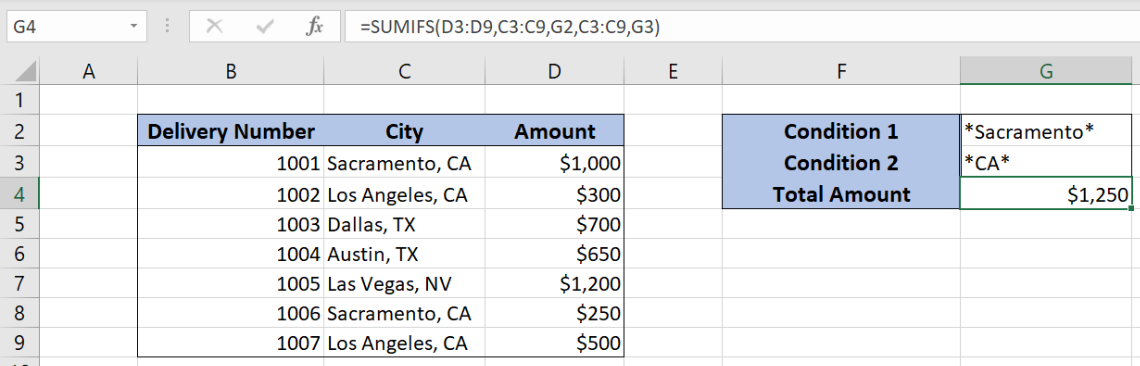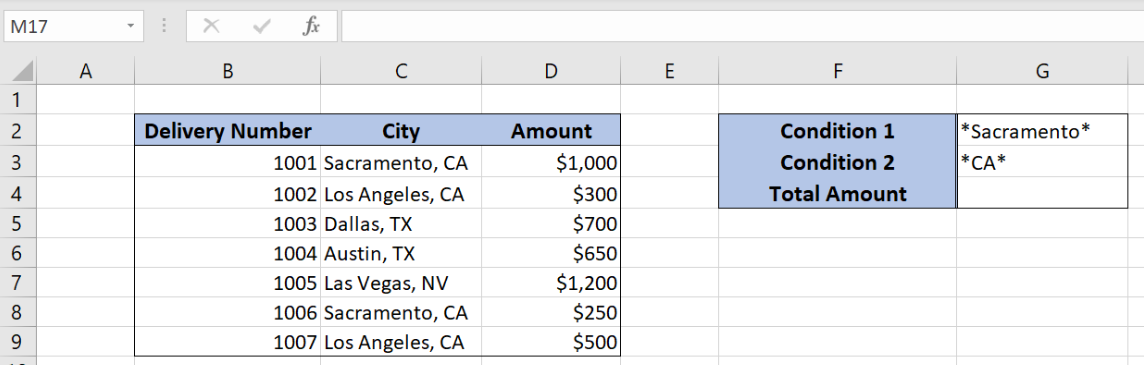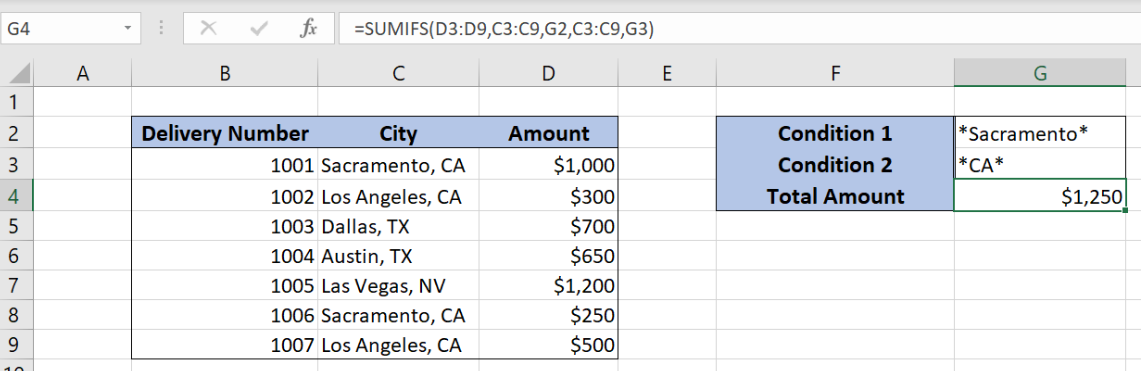Get instant live expert help with Excel or Google Sheets“My Excelchat expert helped me in less than 20 minutes, saving me what would have been 5 hours of work!”

#### Post your problem and you’ll get Expert help in seconds.

Your message must be at least 40 characters
Our professional Expert are available now. Your privacy is guaranteed.

# Sum if cells contain both x and y

Excel allows us to sum all values from a table that contain both x and y by using the SUMIFS function. This step by step tutorial will assist all levels of Excel users in summing values from a table with two conditions.Figure 1. The final result of the SUMIFS function

## Syntax of the SUMIFS formula

`=SUMIFS(sum_range, criteria_range1, criteria1, criteria_range2, criteria2)`

The parameters of the SUMIFS function are:

• sum_range – a range with values which we want to sum
• criteria_range1 – a range where we want to set our first condition
• criteria1 – the first condition for summing the values
• criteria_range2 – a range where we want to set our second condition
• criteria2 – the second condition for summing the values

## Setting up Our Data for the SUMIFS Function

Our table consists of 3 columns: “Delivery Number” (column B), “City” (column C) and “Amount” (column D). In cells G2 and G3, we specify values that we want “City” column to contain. In the cell G4 we want to get a sum for these two conditions.Figure 2. Data that we will use in the SUMIFS example

## Sum Amount if City Contains Both Conditions

In our example, we want to sum all amounts from column D if the cells in column D contains “*Sacramento*” and “*CA*”. The asterisks (*) means that we check if the cell contains the value between them. If we put only the value then, the whole cell must match with that value.

### Formula:

`=SUMIFS(D3:D9, C3:C9, G2, C3:C9, G3)`

The sum_range is D3:D9. Both criteria ranges are C3:C9 (“City” column) Criteria1 is G2 (“*Sacramento*”). Criteria2 is G3 (“*CA*”).

To apply the SUMIFS function, we need to follow these steps:

• Select cell G4 and click on it
• Insert the formula: `=SUMIFS(D3:D9, C3:C9, G2, C3:C9, G3)`
• Press enterFigure 3. Using the SUMIFS function to sum values containing both conditions

In this example, we get all amounts which have “Sacramento” and “CA” in “City”. As you can see, rows 3 and 8 (Sacramento, CA) meet both conditions, so correspondings amounts are summed (\$1,000 and \$250). Finally, the sum in the cell G4 is \$1,250.

Most of the time, the problem you will need to solve will be more complex than a simple application of a formula or function. If you want to save hours of research and frustration, try our live Excelchat service! Our Excel Experts are available 24/7 to answer any Excel question you may have. We guarantee a connection within 30 seconds and a customized solution within 20 minutes.

### Did this post not answer your question? Get a solution from connecting with the expert.Another blog reader asked this question today on Excelchat:
Solution examplesI am trying to utilize a complicated sumif or sumifs formula that can pull a total amount from a date and a drop down box from another tab.
Solved by E. B. in 15 minsI have a spreadsheet that totals invoices. A bunch of cells at the bottom of the spreadsheet calculates totals based on which department the invoice is charged to. I'd like to add a function where it also considers the date (so if it's an invoice in January, it goes to a January total, February, etc.). But I can't figure out how to write it properly...
Solved by K. J. in 27 minsIf I want to write a formula that states" "If this name shows up K24, and this word shows up in J24 pull this number from M24" I can creat that formula - however I need to drag this down so it pulls from row 24 - 60 - is there way to do this?
Solved by F. D. in 40 minsamending a formula so that there is another criteria and if it does not meet this criteria then we stick with the original criteria
Solved by A. B. in 40 minsSo im trying to use a sumif formula, but i need it to not look for a range for one specific criteria but instead look for what one specific field says. Example Below =sum(sumifs(QuantityMC,TeamsMC,A6,TypeMC,B2)) The above formula works as on the specific MC sheet an entire column is dedicated for "TypeMC". I want to be able to delete this column and have "TypeMC" to be a single field as it will be the same for every item on that sheet but may differ on a different sheet.
Solved by M. H. in 45 mins## Subscribe to Excelchat.coAnother blog reader asked this question today on Excelchat: